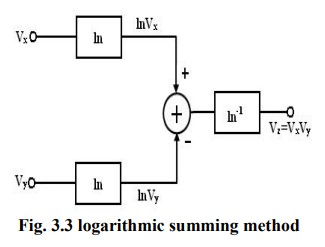Home | | Linear Integrated Circuits | Analog Multipliers

# Analog Multipliers

There are various methods available for performing analog multiplication. Four of such techniques, namely, 1. Logarithmic summing technique 2. Pulse height/width modulation Technique 3. Variable trans conductance Technique 4. Multiplication using Gilbert cell and 5 Multiplication using variable trans conductance technique.

Analog Multipliers:

A multiple produces an output V0 which is proportional to the product of two inputs Vx and Vy. V0 = KVxVy where K is the scaling factor = (1/10) V-1.

There are various methods available for performing analog multiplication. Four of such techniques, namely,

1.        Logarithmic summing technique

2.        Pulse height/width modulation Technique

3.        Variable trans conductance Technique

4.        Multiplication using Gilbert cell and

5       Multiplication using variable trans conductance technique.

An actual multiplier has its output voltage V0 defined bywhere φx and φy are the offsets associated with signals Vx and Vy, ε is the error signal associated with K and φ0 is the offset voltage of the multiplier output.

## Terminologies associated voltage of the multiplier characteristics:

### ·           Accuracy:

This specifies the derivation of the actual output from the ideal output, for any combination of X and Y inputs falling within the permissible operating range of the multiplier.

### ·           Linearity:

This defines the accuracy of the multiplier. The Linearity Error can be defined as the maximum absolute derivation of the error surface. This linearity error imposes a lower limit on the multiplier accuracy.The figure shows the response of the output as a function of one input voltage Vx when the other Vy is assumed constant. It represents the maximum percentage derivation from the ideal straight line output. An error surface is formed by plotting the output for different combinations of X and Y inputs.

### ·           Square law accuracy:

The Square – law curve is obtained with the X and Y inputs connected together and applied with the same input signal. The maximum derivation of the output voltage from an ideal square –law curve expresses the squaring mode accuracy.### ·           Bandwidth:

The Bandwidth indicates the operating capability of an analog multiplier at higher frequency values. Small signal 3 dB bandwidth defines the frequency f0 at which the output reduces by 3dB from its low frequency value for a constant input voltage. This is identified individually for the X and Y input channels normally.

The transconductance bandwidth represents the frequency at which the transconductance of the multiplier drops by 3dB of its low frequency value. This characteristic defines the application frequency ranges when used for phase detection or AM detection.

The quadrant defines the applicability of the circuit for bipolar signals at its inputs. First – quadrant device accepts only positive input signals, the two quadrant device accepts one bipolar signal and one unipolar signal and the four quadrant device accepts two bipolar signals.

### ·           Logarithmic summing Technique:

This technique uses the relationship ln Vx + lnVy =ln(VxVy)

As shown in figure the input voltages Vx and Vy are converted to their logarithmic equivalent, which are then added together by a summer. An antilogarithmic converter produces the output      voltage of the summer. The output is given by,

Vz = ln-1(ln(Vx Vy )) = Vx Vy .The relationship between I0 and VBE of the transistor is given by IC = I0e(VBE /VT ) .It is found that the transistor follows the relationship very accurately in the range of 10nA to 100mA. Logarithmic multiplier has low accuracy and high temperature instability. This method is applicable only to positive values of Vx and Vy.

Limitation: this type of multiplier is restricted to one quadrant operation only.

### ·           Pulse Height/ Width Modulation Technique:In this method, the pulse width of a pulse train is made proportional to one input voltage and the pulse amplitude is made proportional to the second input voltage. Therefore, Vx =Kx A, Vy =Ky t, and Vz =KzT where Kx, Ky, Kz are scaling factors. In figure A is the amplitude of the pulse, t is the pulse width and T is the area of=the pulse=. Therefore,The modulated pulse train is passed through an integrated circuit. Therefore, the input of the integrator is proportional to the area of pulse, which in turn is proportional to the product of two input voltages.

Study Material, Lecturing Notes, Assignment, Reference, Wiki description explanation, brief detail
Linear Integrated Circuits : Analog Multiplier and PLL : Analog Multipliers |